### Home > CALC > Chapter 2 > Lesson 2.3.3 > Problem2-127

2-127.
1.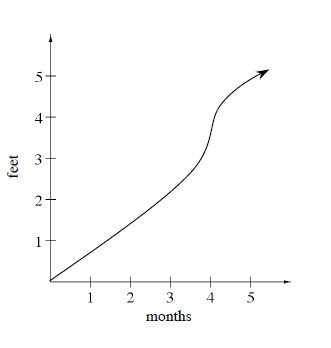Fertilizer needs to be applied during the fastest growth of the plant. Below right is the graph of the growth cycle of a flowering shrub. Homework Help ✎

1. Using complete sentences, write a detailed statement describing the growth of this shrub for 0 ≤ t ≤ 5 months.

2. During which time t should the plant be fertilized?

3. Approximately how fast is the shrub growing at t = 3? How did you get your answer?

4. What is the shrub's average rate of growth over the complete growth cycle? How did you get your answer?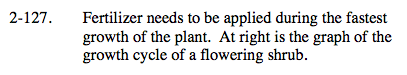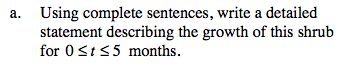Pay attention to variance in the slope of the graph.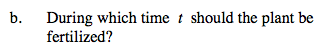When does the fastest growth of the plant occur?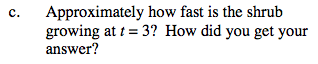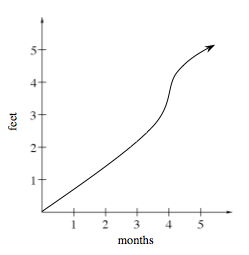Estimate the slope of the secant line at t = 3.

$\approx \frac{3}{4}\text{ feet/month}$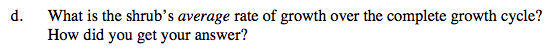The average rate of growth is the slope of the secant line between t = 0 and t = 5.